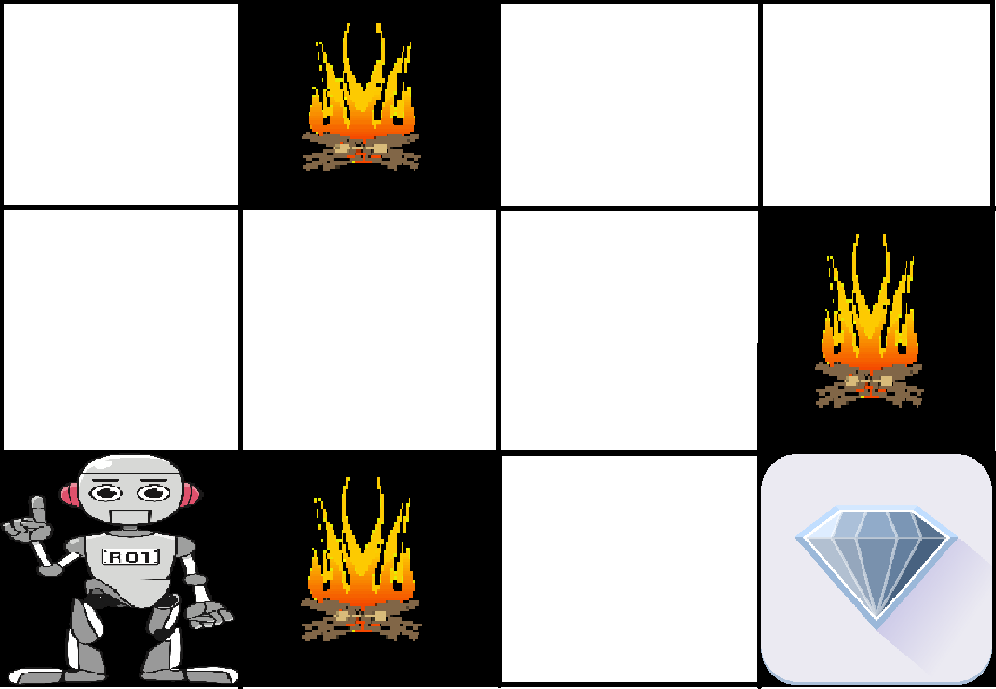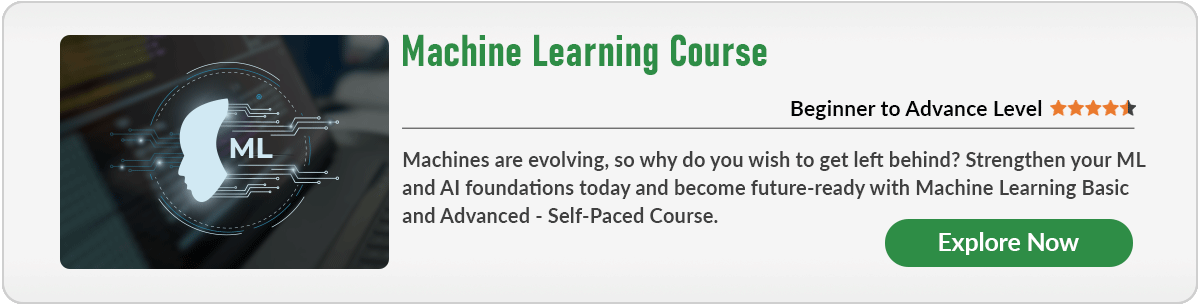Open in App
Not now

# Reinforcement learning

• Difficulty Level : Basic
• Last Updated : 23 Jan, 2023

Reinforcement learning is an area of Machine Learning. It is about taking suitable action to maximize reward in a particular situation. It is employed by various software and machines to find the best possible behavior or path it should take in a specific situation. Reinforcement learning differs from supervised learning in a way that in supervised learning the training data has the answer key with it so the model is trained with the correct answer itself whereas in reinforcement learning, there is no answer but the reinforcement agent decides what to do to perform the given task. In the absence of a training dataset, it is bound to learn from its experience.

Example: The problem is as follows: We have an agent and a reward, with many hurdles in between. The agent is supposed to find the best possible path to reach the reward. The following problem explains the problem more easily.The above image shows the robot, diamond, and fire. The goal of the robot is to get the reward that is the diamond and avoid the hurdles that are fired. The robot learns by trying all the possible paths and then choosing the path which gives him the reward with the least hurdles. Each right step will give the robot a reward and each wrong step will subtract the reward of the robot. The total reward will be calculated when it reaches the final reward that is the diamond.
Main points in Reinforcement learning –

• Input: The input should be an initial state from which the model will start
• Output: There are many possible outputs as there are a variety of solutions to a particular problem
• Training: The training is based upon the input, The model will return a state and the user will decide to reward or punish the model based on its output.
• The model keeps continues to learn.
• The best solution is decided based on the maximum reward.Difference between Reinforcement learning and Supervised learning:

Types of Reinforcement: There are two types of Reinforcement:

1. Positive –
Positive Reinforcement is defined as when an event, occurs due to a particular behavior, increases the strength and the frequency of the behavior. In other words, it has a positive effect on behavior.
• Maximizes Performance
• Sustain Change for a long period of time
• Too much Reinforcement can lead to an overload of states which can diminish the results
2. Negative –
Negative Reinforcement is defined as strengthening of behavior because a negative condition is stopped or avoided.
• Increases Behavior
• Provide defiance to a minimum standard of performance
• It Only provides enough to meet up the minimum behavior

Various Practical applications of Reinforcement Learning –

• RL can be used in robotics for industrial automation.
• RL can be used in machine learning and data processing
• RL can be used to create training systems that provide custom instruction and materials according to the requirement of students.

RL can be used in large environments in the following situations:

1. A model of the environment is known, but an analytic solution is not available;
2. Only a simulation model of the environment is given (the subject of simulation-based optimization)
3. The only way to collect information about the environment is to interact with it.

PY

## Python3

 `import` `gym``import` `numpy as np` `# Define the Q-table and learning rate``q_table ``=` `np.zeros((state_size, action_size))``alpha ``=` `0.8``gamma ``=` `0.95` `# Train the Q-Learning algorithm``for` `episode ``in` `range``(num_episodes):``    ``state ``=` `env.reset()``    ``done ``=` `False``    ``while` `not` `done:``        ``# Choose an action``        ``action ``=` `np.argmax(q_table[state, :] ``+` `np.random.randn(``1``, action_size) ``*` `(``1.` `/` `(episode ``+` `1``)))` `        ``# Take the action and observe the new state and reward``        ``next_state, reward, done, _ ``=` `env.step(action)` `        ``# Update the Q-table``        ``q_table[state, action] ``=` `(``1` `-` `alpha) ``*` `q_table[state, action] ``+` `alpha ``*` `(reward ``+` `gamma ``*` `np.``max``(q_table[next_state, :]))` `        ``state ``=` `next_state` `# Test the trained Q-Learning algorithm``state ``=` `env.reset()``done ``=` `False``while` `not` `done:``    ``# Choose an action``    ``action ``=` `np.argmax(q_table[state, :])` `    ``# Take the action``    ``state, reward, done, _ ``=` `env.step(action)``    ``env.render()`

### GeeksforGeeks Courses

My Personal Notes arrow_drop_up